### Altair SilhouetteHover over the thumbnail for a full-size version.

Author ]{NO3 altair assassin assassins author:]{no3 bitesized creed n-art nart nonplayable rated silhouette two-tone 2007-11-08 5 by 56 people. \$Altair Silhouette#]{NO3#none#00000000000000000000000000000000000000000000000000000000000000000000000000000000000000000000000000000000000000000000000000000000000000000000000000000000000000000000000000000000000000000000000000000000000000000000000000000000000000000000000000000000000000000000000000000000000000000000000000000000000000000000000000000000000000000000000000000000000000000000000000000000000000000000000000000000000000000000000000000000000000000000000000000000000000000000000000000000000000000000000000000000000000000000000000000000000000000000000000000000000000000000000000000000000000000000000000000000000000000000000000000000000000000000000000000000000000000000000000000000000000000000000000000000000000000000000000000000000000000|12^234,138!12^234,144!12^234,150!12^234,156!12^234,162!12^239,163!12^244,163!12^250,162!12^252,160!12^256,159!12^259,157!12^260,153!12^258,149!12^254,146!12^250,145!12^249,141!12^247,138!12^243,135!12^238,135!12^237,141!12^237,145!12^237,149!12^250,165!12^250,168!12^248,172!12^250,176!12^255,179!12^260,178!12^264,176!12^269,176!12^273,176!12^278,177!12^266,178!12^259,181!12^253,184!12^257,189!12^263,191!12^269,189!12^272,187!12^274,186!12^278,188!12^283,191!12^286,189!12^264,149!12^284,176!12^284,171!12^289,178!12^291,182!12^290,187!12^289,193!12^290,198!12^288,202!12^286,206!12^281,210!12^277,208!12^273,211!12^268,209!12^266,206!12^265,201!12^268,197!12^274,194!12^278,193!12^273,190!12^267,194!12^258,198!12^264,198!12^258,192!12^270,150!12^274,152!12^278,157!12^283,163!12^283,166!12^240,174!12^246,174!12^246,180!12^240,180!12^246,168!12^240,168!12^234,168!12^234,174!12^234,180!12^246,192!12^252,192!12^252,198!12^246,198!12^252,186!12^246,186!12^240,186!12^240,192!12^240,198!12^252,210!12^258,210!12^258,216!12^252,216!12^258,204!12^252,204!12^246,204!12^246,210!12^246,216!12^270,222!12^276,222!12^276,228!12^270,228!12^276,216!12^270,216!12^264,216!12^264,222!12^264,228!12^288,222!12^294,222!12^294,228!12^288,228!12^294,216!12^288,216!12^282,216!12^282,222!12^282,228!12^222,126!12^228,126!12^228,132!12^222,132!12^228,120!12^222,120!12^216,120!12^216,126!12^216,132!12^204,126!12^210,126!12^210,132!12^204,132!12^210,120!12^204,120!12^198,120!12^198,126!12^198,132!12^234,132!12^234,126!12^234,120!12^240,120!12^240,126!12^240,132!12^246,132!12^246,126!12^252,126!12^246,120!12^252,132!12^264,210!12^264,204!12^252,138!12^258,138!12^258,132!12^264,138!12^270,138!12^264,132!12^258,126!12^258,144!12^264,144!12^270,144!12^276,144!12^276,150!12^282,150!12^282,156!12^288,156!12^288,162!12^294,162!12^294,168!12^288,168!12^288,174!12^294,174!12^294,180!12^294,186!12^294,192!12^294,198!12^294,204!12^294,210!12^288,210!12^282,210!12^288,204!12^300,168!12^300,174!12^300,180!12^300,186!12^300,192!12^300,198!12^300,210!12^300,204!12^300,216!12^258,222!12^252,222!12^270,234!12^276,234!12^282,234!12^289,233!12^298,221!12^302,208!12^303,202!12^303,198!12^286,150!12^280,144!12^275,138!12^269,133!12^262,128!12^253,122!12^249,121!12^240,117!12^234,114!12^228,114!12^222,114!12^216,114!12^210,114!12^204,114!12^198,114!12^192,114!12^187,116!12^182,117!12^183,123!12^186,127!12^189,133!12^195,129!12^192,124!12^192,120!12^189,120!12^228,138!12^228,144!12^228,150!12^228,156!12^228,168!12^228,162!12^228,174!12^228,180!12^234,186!12^240,204!12^240,210!12^234,192!12^234,198!12^228,186!12^222,138!12^216,138!12^210,138!12^204,138!12^198,138!12^216,150!12^216,144!12^224,147!12^226,143!12^222,156!12^216,156!12^210,150!12^210,144!12^204,144!12^201,144!12^204,150!12^202,149!12^211,153!12^208,153!12^222,168!12^222,162!12^220,163!12^206,160!12^204,156!12^208,165!12^210,170!12^216,174!12^220,174!12^222,174!12^222,180!12^219,181!12^224,188!12^228,192!12^231,198!12^234,204!12^234,210!12^239,216!12^244,218!12^249,225!12^259,229!12^262,234!12^270,240!12^264,234!12^266,240!12^282,240!12^285,238!12^279,239!12^242,81!12^238,78!12^233,78!12^225,74!12^227,78!12^240,66!12^242,63!12^249,62!12^252,59!12^254,53!12^256,49!12^260,47!12^267,46!12^270,45!12^272,51!12^273,56!12^275,61!12^275,66!12^277,73!12^275,69!12^280,78!12^285,77!12^288,77!12^286,82!12^287,89!12^292,88!12^283,86!12^293,85!12^295,81!12^299,81!12^300,76!12^303,81!12^304,85!12^305,91!12^305,96!12^301,93!12^296,92!12^300,90!12^300,84!12^297,87!12^294,97!12^296,103!12^288,96!12^290,91!12^245,68!12^249,71!12^253,73!12^257,75!12^261,75!12^264,78!12^268,82!12^271,85!12^275,87!12^277,91!12^280,95!12^284,99!12^289,102!12^293,105!12^294,109!12^296,113!12^300,117!12^296,108!12^288,96!12^282,90!12^276,84!12^282,84!12^276,78!12^270,78!12^270,72!12^270,66!12^270,60!12^270,54!12^264,54!12^264,48!12^270,48!12^258,54!12^264,60!12^264,66!12^264,72!12^258,66!12^258,60!12^252,66!12^258,72!12^302,125!12^304,130!12^311,131!12^311,127!12^311,122!12^312,118!12^315,118!12^315,123!12^317,130!12^316,135!12^316,141!12^316,146!12^316,151!12^315,157!12^316,162!12^317,167!12^312,150!12^311,146!12^311,141!12^309,136!12^307,133!12^315,139!12^321,118!12^321,114!12^320,109!12^325,116!12^326,121!12^320,102!12^319,97!12^316,89!12^330,134!12^331,140!12^331,145!12^331,151!12^330,158!12^329,163!12^329,167!12^328,172!12^327,178!12^325,184!12^333,142!12^336,143!12^339,137!12^339,132!12^341,144!12^341,149!12^341,155!12^341,161!12^340,167!12^340,173!12^339,179!12^338,183!12^335,188!12^342,180!12^346,176!12^351,175!12^351,170!12^347,166!12^350,161!12^353,156!12^352,150!12^352,144!12^352,138!12^347,151!12^347,156!12^348,162!12^342,168!12^346,170!12^343,172!12^365,175!12^360,177!12^356,181!12^352,184!12^348,187!12^345,190!12^343,194!12^339,198!12^288,246!12^290,250!12^285,253!12^282,250!12^291,248!12^295,248!12^298,245!12^300,241!12^304,238!12^308,234!12^311,230!12^314,227!12^317,222!12^273,287!12^269,287!12^266,290!12^262,294!12^261,299!12^254,304!12^256,302!12^300,266!12^303,263!12^307,260!12^311,257!12^315,253!12^320,248!12^325,244!12^316,251!12^315,260!12^317,264!12^317,267!12^307,270!12^309,268!12^321,261!12^326,258!12^330,255!12^333,251!12^335,247!12^338,243!12^342,239!12^345,236!12^351,231!12^348,233!12^329,241!12^333,236!12^336,231!12^340,228!12^334,227!12^328,229!12^324,232!12^319,236!12^315,238!12^312,240!12^308,245!12^330,224!12^333,219!12^336,217!12^339,211!12^344,207!12^348,201!12^351,197!12^355,194!12^360,188!12^358,191!12^373,184!12^368,188!12^363,194!12^360,196!12^356,199!12^350,202!12^347,205!12^341,218!12^345,214!12^350,212!12^335,223!12^353,208!12^359,207!12^362,204!12^366,200!12^370,199!12^374,197!12^381,191!12^377,194!12^369,201!12^366,204!12^362,207!12^342,230!12^342,234!12^336,234!12^336,240!12^330,246!12^330,252!12^324,252!12^324,246!12^318,258!12^318,258!12^318,252!12^324,258!12^348,228!12^350,224!12^353,222!12^356,220!12^361,217!12^365,214!12^354,227!12^358,224!12^362,220!12^365,216!12^369,215!12^371,212!12^375,211!12^429,189!12^432,185!12^436,182!12^439,180!12^432,182!12^426,184!12^419,187!12^414,190!12^408,192!12^403,195!12^401,197!12^398,199!12^394,201!12^392,203!12^388,205!12^388,209!12^386,206!12^384,208!12^380,209!12^383,213!12^379,215!12^376,218!12^373,222!12^370,225!12^367,229!12^362,234!12^358,239!12^351,246!12^348,250!12^344,253!12^408,360!12^414,360!12^414,366!12^408,366!12^414,354!12^408,354!12^402,354!12^402,360!12^402,366!12^426,360!12^432,360!12^432,366!12^426,366!12^432,354!12^426,354!12^420,354!12^420,360!12^420,366!12^426,342!12^432,342!12^432,348!12^426,348!12^432,336!12^426,336!12^420,336!12^420,342!12^420,348!12^408,342!12^414,342!12^414,348!12^408,348!12^414,336!12^408,336!12^402,336!12^402,342!12^402,348!12^408,324!12^414,324!12^414,330!12^408,330!12^414,318!12^408,318!12^402,318!12^402,324!12^402,330!12^390,324!12^396,324!12^396,330!12^390,330!12^396,318!12^390,318!12^384,318!12^384,324!12^384,330!12^390,342!12^396,342!12^396,348!12^390,348!12^396,336!12^390,336!12^384,336!12^384,342!12^384,348!12^390,360!12^396,360!12^396,366!12^390,366!12^396,354!12^390,354!12^384,354!12^384,360!12^384,366!12^444,360!12^450,360!12^450,366!12^444,366!12^450,354!12^444,354!12^438,354!12^438,360!12^438,366!12^444,342!12^450,342!12^450,348!12^444,348!12^450,336!12^444,336!12^438,336!12^438,342!12^438,348!12^426,324!12^432,324!12^432,330!12^426,330!12^432,318!12^426,318!12^420,318!12^420,324!12^420,330!12^402,306!12^408,306!12^408,312!12^402,312!12^408,300!12^402,300!12^396,300!12^396,306!12^396,312!12^444,378!12^450,378!12^450,384!12^444,384!12^450,372!12^444,372!12^438,372!12^438,378!12^438,384!12^426,378!12^432,378!12^432,384!12^426,384!12^432,372!12^426,372!12^420,372!12^420,378!12^420,384!12^408,378!12^414,378!12^414,384!12^408,384!12^414,372!12^408,372!12^402,372!12^402,378!12^402,384!12^390,378!12^396,378!12^396,384!12^390,384!12^396,372!12^390,372!12^384,372!12^384,378!12^384,384!12^372,372!12^378,372!12^378,378!12^372,378!12^378,366!12^372,366!12^366,366!12^366,372!12^366,378!12^354,366!12^360,366!12^360,372!12^354,372!12^360,360!12^354,360!12^348,360!12^348,366!12^348,372!12^336,360!12^342,360!12^342,366!12^336,366!12^342,354!12^336,354!12^330,354!12^330,360!12^330,366!12^318,354!12^324,354!12^324,360!12^318,360!12^324,348!12^318,348!12^312,348!12^312,354!12^312,360!12^300,342!12^306,342!12^306,348!12^300,348!12^306,336!12^300,336!12^294,336!12^294,342!12^294,348!12^444,396!12^450,396!12^450,402!12^444,402!12^450,390!12^444,390!12^438,390!12^438,396!12^438,402!12^462,396!12^468,396!12^468,402!12^462,402!12^468,390!12^462,390!12^456,390!12^456,396!12^456,402!12^444,414!12^450,414!12^450,420!12^444,420!12^450,408!12^444,408!12^438,408!12^438,414!12^438,420!12^444,432!12^450,432!12^450,438!12^444,438!12^450,426!12^444,426!12^438,426!12^438,432!12^438,438!12^426,426!12^432,426!12^432,432!12^426,432!12^432,420!12^426,420!12^420,420!12^420,426!12^420,432!12^408,420!12^414,420!12^414,426!12^408,426!12^414,414!12^408,414!12^402,414!12^402,420!12^402,426!12^462,438!12^468,438!12^468,444!12^462,444!12^468,432!12^462,432!12^456,432!12^456,438!12^456,444!12^468,456!12^474,456!12^474,462!12^468,462!12^474,450!12^468,450!12^462,450!12^462,456!12^462,462!12^480,408!12^486,408!12^486,414!12^480,414!12^486,402!12^480,402!12^474,402!12^474,408!12^474,414!12^384,312!12^390,312!12^390,300!12^390,306!12^390,294!12^389,287!12^389,283!12^388,290!12^392,292!12^394,296!12^400,296!12^405,296!12^408,291!12^409,283!12^412,293!12^414,300!12^410,296!12^414,306!12^414,312!12^420,312!12^420,306!12^426,312!12^432,312!12^378,312!12^378,318!12^378,324!12^378,330!12^378,336!12^378,342!12^378,348!12^378,354!12^378,360!12^372,360!12^366,360!12^372,354!12^366,354!12^360,354!12^354,354!12^348,354!12^368,350!12^363,349!12^357,347!12^354,348!12^352,344!12^348,344!12^343,344!12^338,342!12^332,342!12^327,340!12^322,338!12^317,337!12^311,335!12^304,331!12^301,329!12^294,327!12^300,330!12^348,348!12^342,348!12^336,348!12^330,348!12^330,342!12^336,342!12^324,342!12^318,342!12^312,342!12^312,336!12^374,342!12^368,338!12^364,331!12^366,334!12^361,327!12^358,322!12^353,321!12^350,320!12^347,317!12^343,315!12^339,313!12^352,316!12^354,317!12^363,324!12^367,328!12^373,325!12^372,330!12^372,336!12^373,317!12^370,312!12^368,306!12^365,300!12^365,296!12^365,291!12^365,287!12^366,278!12^366,282!12^366,297!12^371,301!12^373,307!12^382,304!12^381,308!12^381,301!12^379,297!12^378,293!12^377,288!12^376,284!12^386,269!12^387,263!12^386,265!12^432,390!12^432,396!12^426,396!12^426,390!12^414,390!12^420,390!12^420,396!12^414,396!12^408,396!12^408,390!12^402,390!12^396,390!12^432,408!12^432,402!12^426,402!12^432,414!12^426,414!12^420,414!12^428,412!12^422,401!12^417,400!12^413,400!12^404,397!12^400,394!12^393,391!12^389,390!12^383,389!12^378,388!12^378,384!12^372,384!12^368,383!12^362,383!12^360,378!12^354,378!12^359,380!12^348,378!12^342,378!12^342,372!12^336,372!12^330,372!12^324,372!12^324,366!12^318,366!12^318,372!12^312,363!12^338,375!12^469,414!12^462,414!12^461,420!12^461,424!12^465,427!12^469,429!12^475,416!12^480,417!12^487,418!12^456,426!12^456,420!12^456,414!12^456,408!12^462,408!12^468,408!12^493,417!12^498,418!12^500,413!12^503,409!12^505,405!12^508,403!12^503,401!12^498,401!12^493,401!12^492,408!12^492,414!12^498,414!12^498,408!12^500,404!12^495,404!12^492,404!12^489,397!12^488,393!12^484,390!12^478,387!12^473,383!12^474,390!12^474,396!12^480,396!12^486,396!12^480,390!12^468,384!12^462,384!12^456,384!12^456,378!12^462,378!12^467,378!12^465,373!12^456,372!12^462,372!12^468,368!12^468,363!12^471,363!12^484,364!12^485,369!12^488,371!12^482,368!12^479,366!12^468,357!12^468,350!12^462,366!12^456,366!12^456,360!12^462,360!12^462,354!12^456,354!12^456,348!12^456,342!12^462,342!12^462,348!12^467,345!12^455,335!12^438,330!12^438,324!12^438,318!12^444,318!12^444,324!12^444,330!12^459,338!12^455,330!12^461,330!12^465,333!12^469,338!12^473,339!12^477,342!12^479,346!12^484,349!12^487,352!12^491,355!12^494,360!12^498,365!12^499,370!12^501,374!12^513,399!12^511,395!12^508,391!12^506,386!12^504,382!12^503,379!12^518,402!12^522,406!12^526,407!12^531,408!12^538,409!12^541,414!12^444,306!12^450,306!12^450,312!12^444,312!12^450,300!12^444,300!12^438,300!12^438,306!12^438,312!12^444,288!12^450,288!12^450,294!12^444,294!12^450,282!12^444,282!12^438,282!12^438,288!12^438,294!12^462,306!12^468,306!12^468,312!12^462,312!12^468,300!12^462,300!12^456,300!12^456,306!12^456,312!12^480,324!12^486,324!12^486,330!12^480,330!12^486,318!12^480,318!12^474,318!12^474,324!12^474,330!12^498,342!12^504,342!12^504,348!12^498,348!12^504,336!12^498,336!12^492,336!12^492,342!12^492,348!12^510,360!12^516,360!12^516,366!12^510,366!12^516,354!12^510,354!12^504,354!12^504,360!12^504,366!12^516,378!12^522,378!12^522,384!12^516,384!12^522,372!12^516,372!12^510,372!12^510,378!12^510,384!12^534,396!12^540,396!12^540,402!12^534,402!12^540,390!12^534,390!12^528,390!12^528,396!12^528,402!12^480,306!12^486,306!12^486,312!12^480,312!12^486,300!12^480,300!12^474,300!12^474,306!12^474,312!12^444,270!12^450,270!12^450,276!12^444,276!12^450,264!12^444,264!12^438,264!12^438,270!12^438,276!12^558,378!12^564,378!12^564,384!12^558,384!12^564,372!12^558,372!12^552,372!12^552,378!12^552,384!12^558,360!12^564,360!12^564,366!12^558,366!12^564,354!12^558,354!12^552,354!12^552,360!12^552,366!12^558,342!12^564,342!12^564,348!12^558,348!12^564,336!12^558,336!12^552,336!12^552,342!12^552,348!12^558,324!12^564,324!12^564,330!12^558,330!12^564,318!12^558,318!12^552,318!12^552,324!12^552,330!12^558,306!12^564,306!12^564,312!12^558,312!12^564,300!12^558,300!12^552,300!12^552,306!12^552,312!12^450,330!12^450,324!12^450,318!12^456,318!12^462,318!12^468,318!12^468,324!12^462,324!12^456,324!12^468,330!12^474,336!12^480,336!12^486,336!12^486,342!12^480,342!12^498,354!12^498,360!12^510,348!12^510,342!12^510,336!12^516,348!12^516,342!12^516,336!12^516,330!12^511,332!12^513,327!12^516,322!12^518,326!12^519,330!12^520,338!12^520,343!12^525,345!12^530,343!12^532,340!12^534,335!12^535,333!12^536,338!12^534,341!12^526,349!12^529,352!12^531,356!12^533,360!12^529,358!12^523,355!12^522,348!12^522,354!12^520,359!12^522,366!12^526,365!12^527,369!12^533,368!12^530,371!12^528,372!12^528,378!12^528,384!12^531,381!12^535,382!12^540,384!12^534,384!12^545,384!12^548,381!12^549,376!12^545,371!12^535,370!12^542,368!12^546,366!12^540,363!12^540,359!12^541,355!12^541,351!12^544,347!12^546,348!12^546,354!12^546,360!12^546,342!12^516,396!12^522,396!12^522,390!12^516,390!12^510,390!12^522,402!12^492,330!12^492,324!12^514,317!12^510,316!12^508,315!12^508,310!12^507,303!12^507,297!12^509,292!12^511,286!12^511,282!12^509,278!12^505,277!12^504,281!12^506,285!12^505,290!12^503,291!12^505,302!12^503,300!12^498,292!12^507,263!12^514,257!12^511,247!12^516,234!12^523,238!12^500,213!12^496,217!12^497,222!12^488,226!12^497,241!12^490,256!12^489,261!12^486,263!12^489,263!12^490,270!12^490,275!12^491,281!12^491,288!12^489,293!12^487,289!12^488,285!12^483,298!12^481,295!12^479,291!12^477,288!12^475,282!12^471,288!12^467,286!12^463,282!12^463,276!12^460,275!12^457,272!12^456,267!12^456,264!12^456,294!12^462,294!12^468,294!12^474,294!12^474,288!12^468,288!12^462,288!12^456,288!12^456,282!12^462,282!12^456,276!12^456,270!12^546,336!12^546,330!12^540,324!12^546,324!12^546,318!12^540,318!12^540,312!12^546,312!12^546,306!12^540,306!12^540,300!12^546,300!12^546,294!12^540,294!12^540,288!12^546,288!12^546,282!12^540,282!12^540,276!12^546,276!12^546,270!12^546,264!12^540,258!12^535,301!12^538,304!12^535,294!12^534,288!12^532,283!12^535,276!12^536,282!12^538,270!12^538,268!12^551,266!12^553,270!12^544,258!12^544,260!12^554,274!12^551,274!12^552,282!12^552,277!12^552,288!12^552,294!12^558,294!12^558,288!12^564,288!12^564,294!12^557,282!12^563,284!12^569,287!12^572,291!12^570,294!12^570,300!12^570,306!12^570,312!12^576,312!12^576,306!12^575,300!12^573,296!12^433,284!12^434,290!12^434,296!12^434,278!12^432,272!12^431,266!12^429,259!12^433,258!12^435,264!12^451,259!12^446,258!12^444,253!12^443,248!12^441,254!12^440,259!12^438,252!12^432,252!12^426,252!12^426,246!12^426,240!12^426,234!12^432,240!12^432,246!12^437,247!12^439,243!12^441,239!12^438,229!12^438,233!12^434,233!12^430,233!12^429,227!12^429,227!12^434,222!12^434,216!12^432,216!12^431,221!12^426,223!12^426,228!12^422,217!12^423,222!12^423,227!12^423,232!12^423,212!12^424,208!12^425,204!12^427,201!12^429,207!12^431,212!12^428,216!12^415,276!12^414,272!12^412,268!12^417,266!12^421,263!12^423,268!12^425,272!12^420,260!12^421,255!12^418,259!12^416,256!12^414,260!12^410,259!12^410,255!12^407,247!12^409,252!12^414,247!12^412,243!12^412,239!12^413,235!12^414,239!12^408,238!12^406,235!12^409,221!12^413,222!12^412,218!12^412,214!12^407,206!12^578,317!12^581,322!12^583,328!12^586,334!12^570,318!12^576,318!12^576,324!12^570,324!12^570,330!12^570,342!12^570,336!12^576,336!12^576,330!12^582,336!12^582,330!12^588,339!12^582,342!12^576,342!12^588,342!12^591,345!12^570,348!12^576,348!12^582,348!12^588,348!12^593,348!12^278,319!12^282,321!12^284,323!12^289,330!12^280,332!12^283,332!12^276,330!12^275,333!12^279,338!12^279,345!12^279,343!12^275,344!12^271,344!12^244,336!12^242,341!12^240,345!12^238,349!12^237,353!12^241,352!12^241,358!12^284,347!12^287,348!12^292,350!12^298,353!12^302,354!12^306,354!12^306,360!12^299,361!12^293,360!12^290,359!12^287,357!12^284,357!12^278,356!12^275,354!12^272,352!12^299,362!12^304,365!12^307,364!12^288,348!12^288,342!12^288,336!12^282,342!12^282,336!12^249,331!12^249,328!12^258,357!12^262,361!12^267,387!12^266,385!12^261,380!12^258,378!12^255,375!12^253,371!12^242,385!12^243,389!12^244,391!12^275,367!12^278,367!12^283,372!12^286,375!12^290,377!12^294,379!12^296,376!12^290,372!12^295,372!12^301,372!12^306,372!12^312,372!12^313,376!12^318,377!12^322,382!12^329,382!12^334,381!12^338,382!12^343,384!12^346,386!12^350,387!12^354,388!12^350,394!12^344,394!12^340,393!12^336,389!12^331,386!12^331,389!12^348,399!12^353,402!12^357,404!12^360,407!12^363,410!12^368,413!12^372,413!12^376,412!12^382,413!12^386,416!12^391,420!12^396,423!12^407,410!12^402,408!12^398,408!12^392,407!12^386,407!12^382,404!12^379,402!12^376,400!12^375,398!12^370,397!12^366,396!12^361,396!12^354,396!12^360,402!12^366,402!12^356,400!12^372,402!12^372,408!12^366,408!12^378,408!12^376,404!12^384,408!12^390,414!12^396,414!12^392,410!12^386,409!12^396,419!12^473,431!12^477,434!12^483,438!12^486,442!12^486,466!12^486,461!12^486,455!12^486,449!12^486,447!12^474,438!12^474,444!12^481,440!12^480,444!12^480,450!12^480,456!12^480,462!12^486,473!12^490,476!12^495,484!12^492,481!12^498,488!12^491,486!12^485,482!12^485,479!12^480,480!12^480,474!12^480,468!12^474,468!12^474,474!12^468,468!12^477,478!12^469,472!12^463,466!12^456,462!12^456,456!12^456,450!12^450,450!12^450,444!12^450,456!12^444,450!12^444,444!12^452,460!12^445,454!12^438,449!12^442,453!12^438,444!12^433,450!12^433,445!12^432,438!12^426,438!12^420,438!12^414,432!12^411,432!12^416,435!12^425,442!12^429,444!12^293,394!12^289,391!12^284,391!12^286,393!12^282,393!12^279,393!12^274,396!12^269,396!12^258,394!12^256,391!12^252,396!12^254,400!12^576,360!12^582,360!12^582,366!12^576,366!12^582,354!12^576,354!12^570,354!12^570,360!12^570,366!12^576,378!12^582,378!12^582,384!12^576,384!12^582,372!12^576,372!12^570,372!12^570,378!12^570,384!12^594,378!12^600,378!12^600,384!12^594,384!12^600,372!12^594,372!12^588,372!12^588,378!12^588,384!12^612,378!12^618,378!12^618,384!12^612,384!12^618,372!12^612,372!12^606,372!12^606,378!12^606,384!12^594,360!12^600,360!12^600,366!12^594,366!12^600,354!12^594,354!12^588,354!12^588,360!12^588,366!12^570,396!12^576,396!12^576,402!12^570,402!12^576,390!12^570,390!12^564,390!12^564,396!12^564,402!12^588,396!12^594,396!12^594,402!12^588,402!12^594,390!12^588,390!12^582,390!12^582,396!12^582,402!12^552,414!12^558,414!12^558,420!12^552,420!12^558,408!12^552,408!12^546,408!12^546,414!12^546,420!12^546,402!12^546,390!12^546,396!12^549,400!12^553,396!12^559,400!12^558,396!12^558,390!12^552,390!12^600,396!12^600,390!12^606,360!12^612,363!12^616,367!12^606,366!12^612,366!12^623,380!12^623,386!12^628,387!12^631,386!12^629,391!12^629,395!12^625,394!12^622,394!12^617,394!12^611,394!12^609,394!12^606,390!12^606,390!12^612,390!12^618,390!12^564,408!12^564,414!12^546,425!12^548,429!12^551,435!12^554,431!12^559,427!12^553,426!12^565,425!12^564,419!12^561,434!12^560,438!12^563,441!12^565,436!12^570,439!12^571,444!12^568,448!12^571,435!12^577,437!12^582,437!12^585,431!12^586,427!12^580,426!12^577,429!12^572,427!12^569,425!12^577,446!12^575,441!12^580,441!12^585,442!12^585,446!12^581,447!12^587,449!12^589,446!12^594,441!12^590,438!12^590,434!12^591,430!12^590,424!12^590,421!12^586,421!12^579,421!12^575,421!12^570,420!12^570,414!12^570,408!12^582,408!12^576,408!12^576,414!12^586,411!12^581,411!12^591,411!12^596,412!12^599,415!12^597,420!12^588,408!12^594,408!12^601,404!12^608,404!12^606,404!12^615,404!12^621,403!12^627,403!12^600,408!12^606,408!12^612,408!12^618,408!12^624,408!12^630,408!12^631,403!12^606,414!12^600,414!12^600,420!12^606,420!12^612,420!12^612,414!12^594,426!12^594,432!12^594,438!12^600,438!12^600,432!12^600,426!12^554,447!12^554,443!12^552,448!12^569,452!12^567,456!12^567,459!12^574,453!12^577,456!12^581,456!12^584,459!12^577,458!12^589,456!12^590,464!12^586,470!12^579,470!12^575,471!12^571,471!12^567,472!12^563,472!12^560,472!12^556,472!12^555,468!12^543,468!12^546,465!12^543,477!12^542,483!12^540,485!12^545,478!12^618,414!12^624,414!12^630,414!12^642,414!12^636,414!12^636,408!12^635,405!12^643,407!12^642,408!12^648,410!12^655,412!12^659,415!12^664,419!12^668,423!12^672,428!12^676,434!12^678,440!12^673,436!12^668,432!12^662,428!12^665,431!12^657,426!12^653,423!12^648,422!12^643,419!12^639,419!12^648,414!12^648,420!12^654,420!12^654,414!12^660,420!12^660,426!12^666,426!12^633,419!12^632,422!12^636,424!12^642,426!12^647,430!12^652,432!12^657,436!12^664,440!12^667,443!12^670,446!12^673,450!12^677,454!12^680,459!12^682,464!12^678,474!12^675,469!12^669,467!12^672,462!12^675,462!12^673,458!12^667,457!12^666,454!12^663,451!12^662,449!12^658,448!12^655,445!12^652,443!12^649,441!12^645,441!12^642,440!12^637,438!12^636,434!12^639,431!12^642,431!12^636,429!12^633,441!12^636,447!12^642,449!12^647,446!12^624,426!12^630,426!12^630,432!12^624,432!12^630,420!12^624,420!12^618,420!12^618,426!12^618,432!12^624,444!12^630,444!12^630,450!12^624,450!12^630,438!12^624,438!12^618,438!12^618,444!12^618,450!12^606,450!12^612,450!12^612,456!12^606,456!12^612,444!12^606,444!12^600,444!12^600,450!12^600,456!12^606,468!12^612,468!12^612,474!12^606,474!12^612,462!12^606,462!12^600,462!12^600,468!12^600,474!12^594,498!12^600,498!12^600,504!12^594,504!12^600,492!12^594,492!12^588,492!12^588,498!12^588,504!12^612,432!12^612,426!12^606,426!12^606,432!12^606,438!12^612,438!12^594,450!12^594,456!12^594,462!12^594,468!12^594,474!12^594,480!12^588,480!12^582,480!12^584,475!12^578,478!12^572,478!12^567,478!12^563,478!12^571,483!12^567,486!12^563,485!12^581,484!12^577,487!12^577,492!12^582,492!12^582,486!12^588,486!12^594,486!12^600,486!12^600,480!12^582,498!12^576,498!12^570,498!12^565,494!12^560,491!12^555,488!12^554,484!12^552,484!12^556,493!12^557,500!12^554,505!12^558,507!12^562,511!12^564,515!12^564,498!12^558,498!12^558,504!12^564,504!12^570,504!12^576,504!12^582,504!12^570,510!12^564,510!12^576,510!12^582,510!12^588,510!12^594,510!12^600,510!12^618,478!12^625,480!12^630,483!12^636,485!12^643,483!12^641,478!12^636,477!12^632,475!12^627,473!12^621,470!12^617,470!12^632,494!12^624,494!12^621,492!12^616,489!12^616,483!12^620,483!12^627,486!12^624,485!12^632,487!12^635,490!12^672,505!12^670,498!12^671,501!12^669,492!12^667,488!12^666,484!12^669,480!12^674,476!12^677,488!12^681,485!12^685,481!12^685,474!12^647,399!12^653,399!12^656,403!12^661,405!12^664,408!12^668,411!12^671,413!12^673,415!12^675,418!12^677,422!12^678,425!12^669,510!12^667,514!12^667,504!12^663,504!12^666,496!12^664,494!12^658,494!12^654,491!12^651,496!12^652,502!12^656,505!12^658,510!12^662,512!12^658,515!12^654,519!12^655,524!12^661,531!12^658,536!12^653,541!12^648,540!12^647,535!12^649,529!12^653,527!12^649,547!12^649,553!12^647,545!12^644,543!12^641,539!12^635,535!12^632,538!12^635,542!12^630,542!12^629,546!12^631,550!12^634,553!12^637,557!12^640,561!12^645,562!12^650,557!12^647,562!12^640,568!12^645,566!12^640,574!12^638,580!12^636,583!12^568,513!12^546,527!12^551,525!12^555,523!12^561,523!12^567,523!12^573,521!12^574,514!12^571,514!12^578,517!12^566,534!12^570,530!12^568,526!12^573,525!12^562,535!12^553,535!12^554,533!12^550,533!12^547,535!12^546,540!12^541,534!12^536,544!12^536,547!12^532,552!12^537,550!12^541,549!12^558,542!12^562,542!12^560,551!12^566,547!12^571,545!12^571,537!12^544,561!12^547,559!12^551,558!12^555,561!12^552,571!12^553,564!12^552,562!12^558,571!12^562,571!12^558,567!12^559,565!12^565,569!12^566,564!12^568,558!12^565,553!12^569,552!12^575,548!12^576,554!12^574,557!12^572,562!12^571,567!12^568,572!12^581,551!12^580,544!12^584,544!12^586,539!12^591,541!12^591,547!12^590,551!12^588,556!12^588,562!12^587,567!12^583,569!12^576,569!12^581,556!12^573,573!12^578,573!12^583,573!12^592,568!12^597,570!12^602,571!12^607,571!12^612,571!12^617,571!12^618,575!12^613,578!12^607,578!12^603,578!12^598,578!12^592,578!12^589,574!12^582,576!12^588,576!12^588,582!12^582,582!12^576,582!12^576,576!12^570,576!12^570,582!12^564,582!12^558,582!12^552,582!12^546,582!12^594,582!12^600,582!12^612,582!12^606,582!12^618,582!12^624,582!12^630,582!12^630,576!12^624,576!12^588,522!12^594,522!12^594,528!12^588,528!12^594,516!12^588,516!12^582,516!12^582,522!12^582,528!12^606,522!12^612,522!12^612,528!12^606,528!12^612,516!12^606,516!12^600,516!12^600,522!12^600,528!12^612,504!12^618,504!12^618,510!12^612,510!12^618,498!12^612,498!12^606,498!12^606,504!12^606,510!12^624,522!12^630,522!12^630,528!12^624,528!12^630,516!12^624,516!12^618,516!12^618,522!12^618,528!12^642,522!12^648,522!12^648,528!12^642,528!12^648,516!12^642,516!12^636,516!12^636,522!12^636,528!12^630,504!12^636,504!12^636,510!12^630,510!12^636,498!12^630,498!12^624,498!12^624,504!12^624,510!12^642,462!12^648,462!12^648,468!12^642,468!12^648,456!12^642,456!12^636,456!12^636,462!12^636,468!12^624,462!12^630,462!12^630,468!12^624,468!12^630,456!12^624,456!12^618,456!12^618,462!12^618,468!12^660,462!12^666,462!12^666,468!12^660,468!12^666,456!12^660,456!12^654,456!12^654,462!12^654,468!12^654,480!12^660,480!12^660,486!12^654,486!12^660,474!12^654,474!12^648,474!12^648,480!12^648,486!12^618,540!12^624,540!12^624,546!12^618,546!12^624,534!12^618,534!12^612,534!12^612,540!12^612,546!12^618,558!12^624,558!12^624,564!12^618,564!12^624,552!12^618,552!12^612,552!12^612,558!12^612,564!12^600,558!12^606,558!12^606,564!12^600,564!12^606,552!12^600,552!12^594,552!12^594,558!12^594,564!12^600,540!12^606,540!12^606,546!12^600,546!12^606,534!12^600,534!12^594,534!12^594,540!12^594,546!12^576,540!12^576,534!12^576,528!12^576,522!12^570,534!12^582,534!12^588,534!12^582,540!12^630,558!12^630,552!12^630,564!12^636,564!12^636,558!12^636,570!12^636,576!12^624,570!12^630,570!12^642,534!12^630,534!12^642,510!12^642,504!12^642,498!12^642,492!12^648,492!12^642,486!12^648,498!12^648,504!12^648,510!12^654,510!12^654,516!12^612,486!12^606,486!12^606,480!12^612,480!12^612,492!12^606,492!12^642,474!12^636,473!12^672,474!12^666,474!12^666,480!12^654,450!12^648,450!12^360,427!12^364,424!12^366,421!12^370,423!12^376,423!12^382,423!12^388,426!12^391,428!12^396,432!12^400,433!12^404,435!12^407,438!12^412,442!12^416,445!12^420,449!12^424,451!12^428,455!12^431,457!12^434,460!12^444,464!12^439,463!12^449,468!12^452,471!12^455,473!12^459,476!12^454,476!12^453,480!12^448,479!12^454,485!12^444,475!12^443,472!12^438,469!12^434,469!12^431,467!12^429,462!12^444,483!12^447,487!12^452,491!12^457,494!12^462,498!12^467,502!12^471,510!12^474,513!12^475,509!12^477,507!12^480,506!12^483,504!12^497,509!12^502,512!12^504,517!12^504,522!12^503,526!12^503,530!12^504,535!12^507,542!12^509,546!12^513,551!12^512,557!12^509,560!12^509,564!12^506,570!12^503,568!12^499,566!12^493,563!12^490,561!12^496,558!12^502,561!12^506,561!12^490,556!12^485,557!12^480,554!12^474,553!12^469,551!12^467,547!12^463,543!12^459,537!12^455,537!12^453,540!12^451,544!12^448,548!12^453,552!12^457,555!12^462,556!12^449,555!12^444,555!12^440,558!12^437,564!12^441,566!12^446,568!12^451,567!12^455,568!12^463,569!12^468,569!12^473,569!12^479,569!12^485,569!12^477,572!12^474,575!12^476,580!12^249,574!12^250,569!12^245,567!12^239,567!12^239,562!12^238,554!12^238,548!12^237,541!12^237,545!12^237,537!12^236,529!12^236,534!12^236,526!12^236,520!12^237,516!12^237,510!12^238,505!12^238,500!12^232,499!12^236,497!12^238,492!12^239,487!12^245,484!12^241,481!12^249,467!12^249,473!12^252,472!12^261,470!12^261,475!12^257,478!12^248,483!12^253,483!12^266,474!12^271,474!12^266,472!12^275,476!12^281,477!12^287,480!12^291,483!12^288,472!12^291,473!12^296,475!12^301,477!12^305,479!12^310,482!12^296,485!12^300,489!12^305,491!12^305,495!12^306,501!12^312,502!12^317,500!12^318,497!12^316,492!12^315,487!12^310,485!12^316,487!12^322,487!12^307,511!12^304,507!12^300,504!12^295,505!12^295,509!12^294,515!12^297,517!12^304,516!12^308,515!12^441,547!12^436,545!12^433,544!12^428,541!12^422,539!12^417,537!12^411,533!12^414,528!12^420,528!12^426,531!12^432,533!12^437,535!12^443,538!12^448,540!12^448,538!12^434,528!12^429,524!12^417,521!12^420,518!12^420,515!12^423,512!12^430,512!12^435,514!12^440,518!12^445,520!12^446,530!12^443,527!12^451,523!12^455,527!12^455,531!12^480,521!12^477,519!12^485,517!12^485,521!12^490,521!12^495,523!12^499,521!12^327,483!12^330,478!12^329,474!12^327,470!12^325,465!12^325,457!12^320,459!12^318,463!12^314,463!12^307,462!12^303,459!12^298,455!12^293,453!12^289,451!12^284,449!12^281,447!12^286,442!12^288,446!12^297,451!12^296,445!12^299,442!12^301,436!12^306,431!12^306,427!12^305,423!12^304,417!12^249,414!12^253,412!12^258,409!12^263,407!12^269,405!12^274,404!12^280,404!12^277,402!12^282,402!12^289,402!12^294,402!12^299,402!12^304,402!12^289,405!12^292,406!12^286,406!12^296,407!12^301,409!12^301,406!12^305,412!12^282,430!12^284,424!12^285,419!12^279,432!12^282,433!12^272,442!12^261,461!12^264,462!12^278,466!12^282,468!12^225,516!12^225,522!12^222,517!12^221,512!12^218,516!12^309,406!12^315,407!12^320,410!12^325,413!12^332,418!12^328,415!12^336,422!12^340,427!12^343,429!12^344,433!12^344,438!12^350,440!12^355,440!12^359,437!12^362,433!12^364,429!12^318,426!12^324,426!12^324,432!12^318,432!12^324,420!12^318,420!12^312,420!12^312,426!12^312,432!12^318,444!12^324,444!12^324,450!12^318,450!12^324,438!12^318,438!12^312,438!12^312,444!12^312,450!12^336,444!12^342,444!12^342,450!12^336,450!12^342,438!12^336,438!12^330,438!12^330,444!12^330,450!12^372,444!12^378,444!12^378,450!12^372,450!12^378,438!12^372,438!12^366,438!12^366,444!12^366,450!12^372,462!12^378,462!12^378,468!12^372,468!12^378,456!12^372,456!12^366,456!12^366,462!12^366,468!12^354,462!12^360,462!12^360,468!12^354,468!12^360,456!12^354,456!12^348,456!12^348,462!12^348,468!12^336,462!12^342,462!12^342,468!12^336,468!12^342,456!12^336,456!12^330,456!12^330,462!12^330,468!12^354,480!12^360,480!12^360,486!12^354,486!12^360,474!12^354,474!12^348,474!12^348,480!12^348,486!12^372,480!12^378,480!12^378,486!12^372,486!12^378,474!12^372,474!12^366,474!12^366,480!12^366,486!12^390,480!12^396,480!12^396,486!12^390,486!12^396,474!12^390,474!12^384,474!12^384,480!12^384,486!12^390,462!12^396,462!12^396,468!12^390,468!12^396,456!12^390,456!12^384,456!12^384,462!12^384,468!12^390,444!12^396,444!12^396,450!12^390,450!12^396,438!12^390,438!12^384,438!12^384,444!12^384,450!12^408,462!12^414,462!12^414,468!12^408,468!12^414,456!12^408,456!12^402,456!12^402,462!12^402,468!12^408,480!12^414,480!12^414,486!12^408,486!12^414,474!12^408,474!12^402,474!12^402,480!12^402,486!12^426,480!12^432,480!12^432,486!12^426,486!12^432,474!12^426,474!12^420,474!12^420,480!12^420,486!12^426,498!12^432,498!12^432,504!12^426,504!12^432,492!12^426,492!12^420,492!12^420,498!12^420,504!12^408,498!12^414,498!12^414,504!12^408,504!12^414,492!12^408,492!12^402,492!12^402,498!12^402,504!12^390,498!12^396,498!12^396,504!12^390,504!12^396,492!12^390,492!12^384,492!12^384,498!12^384,504!12^372,498!12^378,498!12^378,504!12^372,504!12^378,492!12^372,492!12^366,492!12^366,498!12^366,504!12^354,498!12^360,498!12^360,504!12^354,504!12^360,492!12^354,492!12^348,492!12^348,498!12^348,504!12^336,498!12^342,498!12^342,504!12^336,504!12^342,492!12^336,492!12^330,492!12^330,498!12^330,504!12^336,516!12^342,516!12^342,522!12^336,522!12^342,510!12^336,510!12^330,510!12^330,516!12^330,522!12^354,516!12^360,516!12^360,522!12^354,522!12^360,510!12^354,510!12^348,510!12^348,516!12^348,522!12^372,516!12^378,516!12^378,522!12^372,522!12^378,510!12^372,510!12^366,510!12^366,516!12^366,522!12^390,516!12^396,516!12^396,522!12^390,522!12^396,510!12^390,510!12^384,510!12^384,516!12^384,522!12^408,516!12^414,516!12^414,522!12^408,522!12^414,510!12^408,510!12^402,510!12^402,516!12^402,522!12^444,498!12^450,498!12^450,504!12^444,504!12^450,492!12^444,492!12^438,492!12^438,498!12^438,504!12^462,516!12^468,516!12^468,522!12^462,522!12^468,510!12^462,510!12^456,510!12^456,516!12^456,522!12^480,534!12^486,534!12^486,540!12^480,540!12^486,528!12^480,528!12^474,528!12^474,534!12^474,540!12^498,546!12^504,546!12^504,552!12^498,552!12^504,540!12^498,540!12^492,540!12^492,546!12^492,552!12^276,516!12^282,516!12^282,522!12^276,522!12^282,510!12^276,510!12^270,510!12^270,516!12^270,522!12^258,516!12^264,516!12^264,522!12^258,522!12^264,510!12^258,510!12^252,510!12^252,516!12^252,522!12^258,498!12^264,498!12^264,504!12^258,504!12^264,492!12^258,492!12^252,492!12^252,498!12^252,504!12^276,498!12^282,498!12^282,504!12^276,504!12^282,492!12^276,492!12^270,492!12^270,498!12^270,504!12^258,534!12^264,534!12^264,540!12^258,540!12^264,528!12^258,528!12^252,528!12^252,534!12^252,540!12^276,534!12^282,534!12^282,540!12^276,540!12^282,528!12^276,528!12^270,528!12^270,534!12^270,540!12^288,510!12^288,504!12^288,498!12^288,492!12^294,492!12^294,498!12^300,498!12^300,492!12^288,486!12^294,486!12^282,486!12^276,486!12^270,486!12^264,486!12^258,486!12^252,486!12^258,480!12^264,480!12^276,480!12^270,480!12^282,480!12^246,492!12^246,486!12^240,492!12^240,498!12^246,498!12^246,504!12^246,510!12^246,516!12^246,522!12^246,528!12^246,534!12^246,540!12^240,540!12^240,534!12^246,528!12^240,528!12^240,522!12^240,516!12^240,510!12^240,504!12^336,486!12^342,486!12^342,480!12^342,474!12^336,474!12^336,480!12^330,486!12^318,456!12^312,456!12^306,456!12^306,450!12^301,448!12^306,444!12^306,438!12^300,444!12^312,438!12^312,414!12^306,414!12^306,420!12^310,411!12^318,414!12^330,426!12^330,420!12^330,432!12^336,432!12^336,426!12^342,432!12^348,444!12^354,444!12^360,444!12^360,438!12^360,438!12^360,450!12^354,450!12^348,450!12^366,432!12^366,426!12^372,426!12^372,432!12^378,432!12^378,426!12^384,426!12^384,432!12^390,432!12^408,450!12^402,450!12^402,444!12^402,438!12^408,444!12^414,450!12^420,456!12^426,456!12^426,462!12^420,462!12^420,468!12^426,468!12^438,474!12^438,480!12^438,486!12^444,486!12^456,504!12^456,498!12^462,504!12^450,510!12^444,510!12^444,510!12^438,510!12^450,516!12^444,516!12^420,510!12^426,510!12^432,510!12^462,528!12^468,528!12^474,522!12^474,516!12^498,534!12^492,534!12^492,528!12^498,528!12^486,546!12^480,546!12^474,546!12^468,540!12^468,534!12^462,534!12^486,552!12^480,552!12^504,558!12^510,552!12^324,510!12^324,504!12^324,498!12^330,492!12^324,492!12^318,492!12^318,504!12^312,510!12^306,504!12^312,504!12^318,510!12^312,516!12^318,516!12^324,516!12^324,522!12^318,522!12^312,522!12^306,522!12^300,522!12^294,522!12^288,516!12^288,522!12^312,564!12^312,558!12^312,552!12^312,546!12^312,540!12^318,552!12^324,546!12^324,540!12^324,558!12^324,564!12^336,564!12^336,558!12^336,552!12^336,546!12^336,540!12^342,540!12^348,546!12^348,552!12^348,564!12^348,558!12^360,564!12^354,558!12^354,552!12^354,546!12^360,540!12^366,540!12^372,546!12^372,552!12^372,558!12^366,564!12^384,564!12^390,564!12^396,558!12^390,552!12^396,546!12^390,540!12^384,540!12^396,540!12^396,552!12^396,564!12^384,552!12^384,546!12^390,546!12^390,558!12^384,558!12^378,558!12^378,564!12^372,564!12^378,552!12^378,546!12^378,540!12^372,540!12^366,546!12^360,546!12^360,552!12^360,558!12^366,558!12^366,552!12^354,540!12^348,540!12^354,564!12^342,564!12^342,558!12^342,552!12^342,546!12^330,540!12^330,546!12^330,552!12^324,552!12^330,558!12^330,564!12^318,558!12^318,564!12^318,546!12^318,540!12^312,534!12^312,528!12^318,528!12^318,534!12^324,534!12^294,534!12^300,534!12^300,540!12^294,540!12^300,528!12^294,528!12^288,528!12^288,534!12^288,540!12^294,552!12^300,552!12^300,558!12^294,558!12^300,546!12^294,546!12^288,546!12^288,552!12^288,558!12^276,552!12^282,552!12^282,558!12^276,558!12^282,546!12^276,546!12^270,546!12^270,552!12^270,558!12^258,552!12^264,552!12^264,558!12^258,558!12^264,546!12^258,546!12^252,546!12^252,552!12^252,558!12^258,570!12^264,570!12^264,576!12^258,576!12^264,564!12^258,564!12^252,564!12^252,570!12^252,576!12^276,570!12^282,570!12^282,576!12^276,576!12^282,564!12^276,564!12^270,564!12^270,570!12^270,576!12^294,570!12^300,570!12^300,576!12^294,576!12^300,564!12^294,564!12^288,564!12^288,570!12^288,576!12^408,570!12^414,570!12^414,576!12^408,576!12^414,564!12^408,564!12^402,564!12^402,570!12^402,576!12^408,552!12^414,552!12^414,558!12^408,558!12^414,546!12^408,546!12^402,546!12^402,552!12^402,558!12^426,552!12^432,552!12^432,558!12^426,558!12^432,546!12^426,546!12^420,546!12^420,552!12^420,558!12^426,570!12^432,570!12^432,576!12^426,576!12^432,564!12^426,564!12^420,564!12^420,570!12^420,576!12^408,534!12^414,534!12^414,540!12^408,540!12^414,528!12^408,528!12^402,528!12^402,534!12^402,540!12^330,534!12^336,534!12^336,540!12^330,540!12^336,528!12^330,528!12^324,528!12^324,534!12^324,540!12^348,534!12^354,534!12^354,540!12^348,540!12^354,528!12^348,528!12^342,528!12^342,534!12^342,540!12^366,534!12^372,534!12^372,540!12^366,540!12^372,528!12^366,528!12^360,528!12^360,534!12^360,540!12^384,534!12^390,534!12^390,540!12^384,540!12^390,528!12^384,528!12^378,528!12^378,534!12^378,540!12^396,528!12^396,534!12^306,528!12^306,534!12^306,540!12^306,546!12^306,552!12^306,558!12^306,564!12^246,558!12^246,552!12^246,546!12^240,546!12^240,552!12^240,558!12^246,564!12^312,570!12^318,570!12^318,576!12^312,576!12^318,564!12^312,564!12^306,564!12^306,570!12^306,576!12^330,570!12^336,570!12^336,576!12^330,576!12^336,564!12^330,564!12^324,564!12^324,570!12^324,576!12^348,570!12^354,570!12^354,576!12^348,576!12^354,564!12^348,564!12^342,564!12^342,570!12^342,576!12^366,570!12^372,570!12^372,576!12^366,576!12^372,564!12^366,564!12^360,564!12^360,570!12^360,576!12^384,570!12^390,570!12^390,576!12^384,576!12^390,564!12^384,564!12^378,564!12^378,570!12^378,576!12^396,570!12^396,576!12^438,552!12^444,552!12^438,570!12^438,576!12^444,576!12^450,576!12^444,570!12^450,570!12^456,570!12^462,570!12^462,576!12^456,576!12^468,576!12^474,570# The main charachter from assassins creed. the game looks very promising. cant wait. A quick N-art, about two hours of work. It'll be the last n-art from me until the holidays, (December). rate and comment please. please dont snipe or spam. enjoi.

## Other maps by this author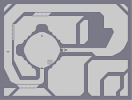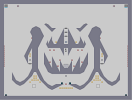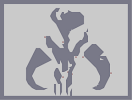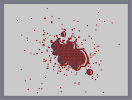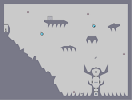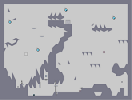KRA Malevolent Death Head Blood Malediction The watch tower Ideas for Cahu

Pages: (0)

5/5

### Awesome... Totally

and utterly awesome.

Neat

### AY...

A FLIPPED VERSION IS EXUBERANCES AVI!!! COL. 5

### It's not the best map ever,

it's not a map... it's art

### 5aved!!!!!!

this is the best map EVER!!!!

GREAT
WOW
MASTER!!!!
F@#KI&**# GOOD!!!1

5/5

### PS3

IS THE TOP OF TECHNOLOGY TODAY

### OOOOOOOOOOOOOOYYYYYAAAAAAAAAAAAA

ALTAIR IS THE BEST

### Awesome!

Like I said: AWESOME!

### Ohhh!..

I see... it's a distraction from your homework! :P

### @ JackPack

i dunno, I used to be really patient. and plus making this kinda stuff is better than doing homework.

### 5aved!

How do you have the patience to do this?

### 5aved

I cant wait for the game to release.

### ooooh

cant wait for this
and this is very good. 4.5/5
PC > 360 >> PS3

faved.

### Su-weet

The image is awesome but as Be-Happy said, the lower part is hard to make out. 4.5/5

Wouldv'e given a 5 but the bottom needs improving.

### i wouldnt load in the editor unless you

have an effing good computer.

yer...

### For the record:

This map has been top rated.

### wow

great use of negative space conjoined with the outer space... 5/5, after some consideration!

by me

### umm

I didn't like the way the hood was formed.
4.5/5 nonetheless

### 5aved

awesome detail. I cant wait till it comes out on pc next yr.
i agree with crank_dat, 360 > ps3;

### 5aved

only because i want this game so badly.

First I need a PS3 :/

### VERY NICE

nice me likey. also xbox 360 kicks PS3 ass

### VERY NICE

nice me likey. also xbox 360 kicks PS3 ass

### WOW

caint make out the lower part oof he body very well though

5aved.